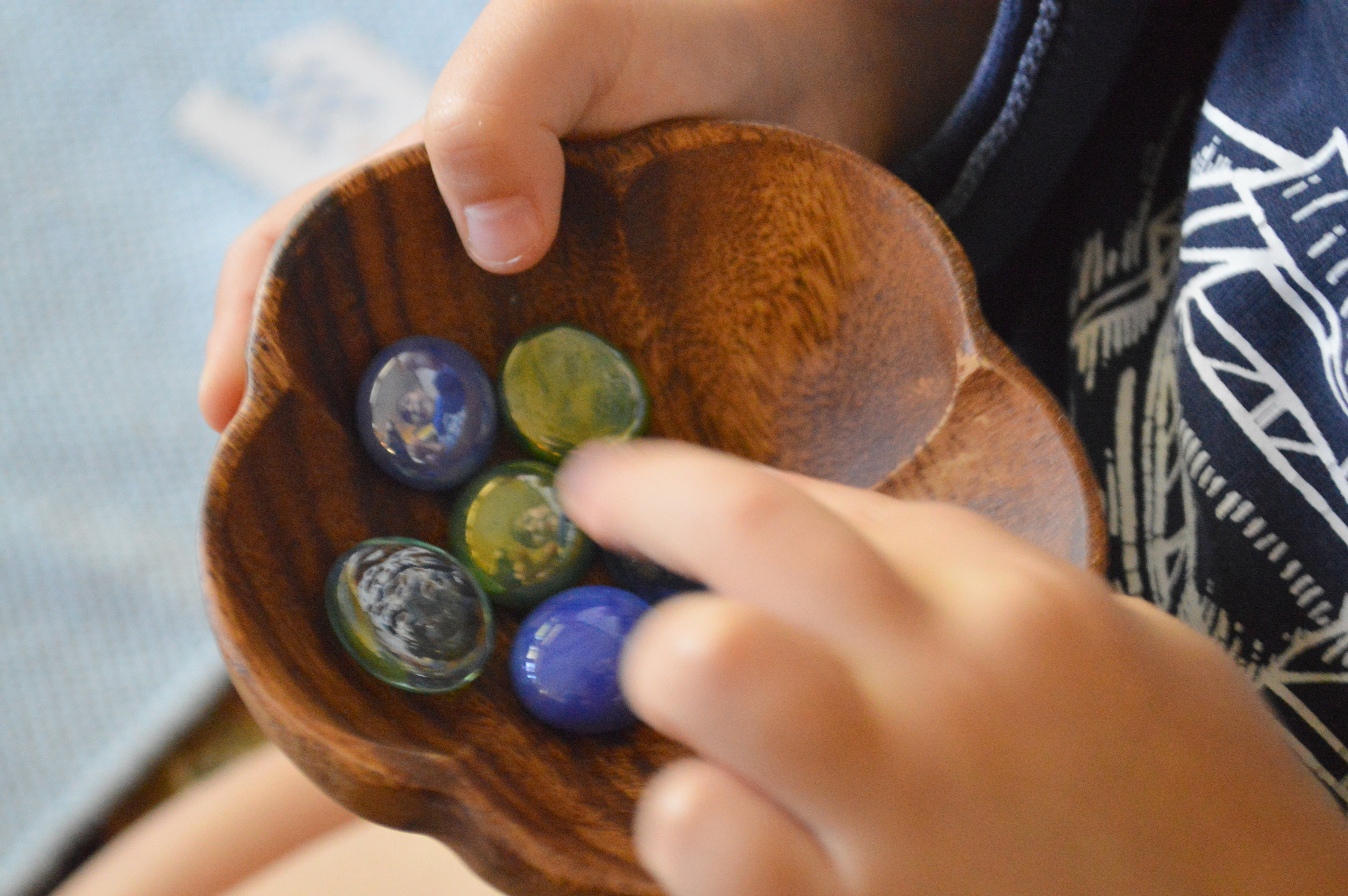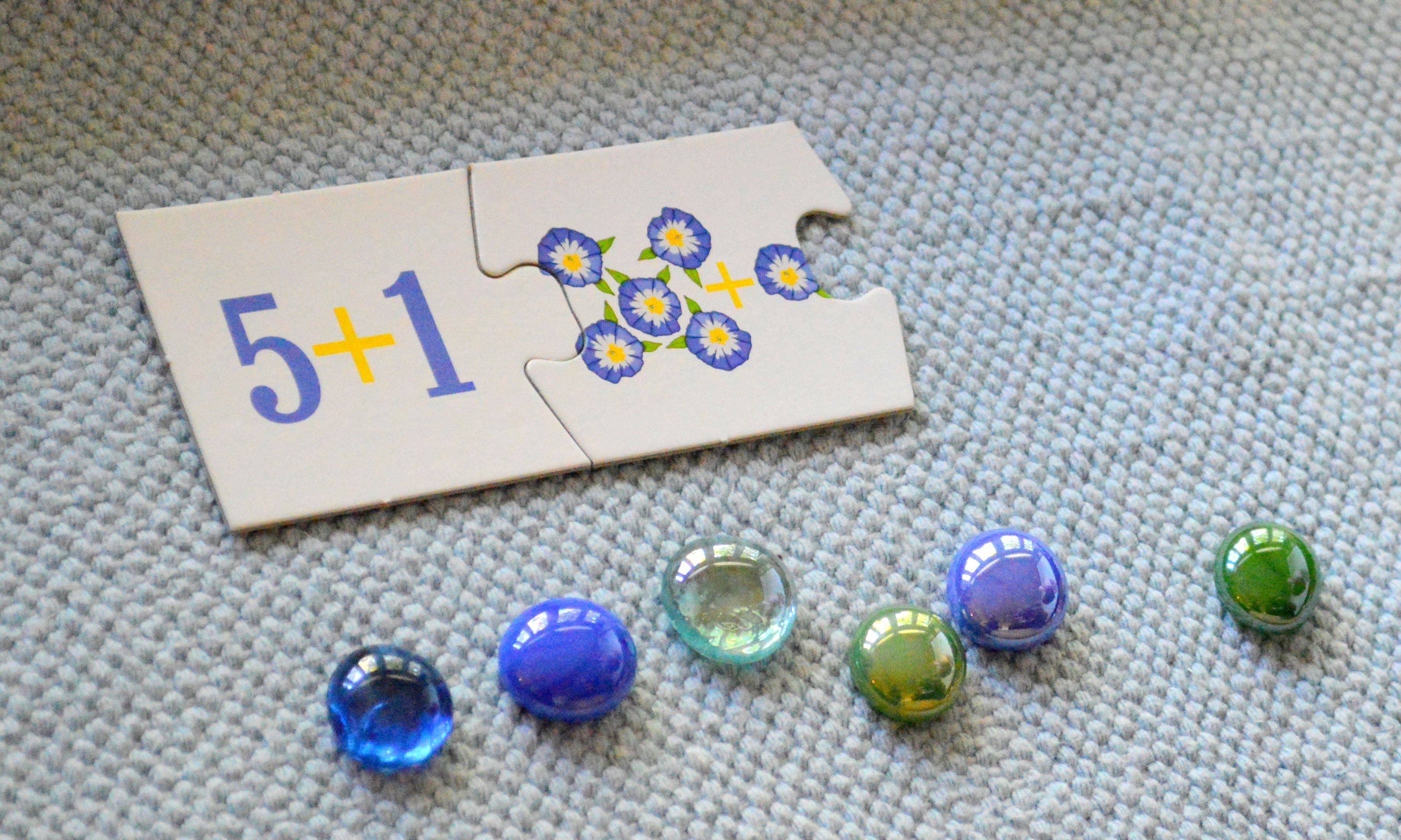When should a toddler be expected to do simple addition with numerals 1-10? If using Montessori methods, such as Addition Strip Board, then probably even before the third birthday. The beauty of Montessori method is that complex abstract concepts are broken down into concrete steps, understandable even to a two year old. If your toddler can count till ten, then he/she can perform such simple additions using counters.

Adrian, being almost three years old, is very familiar with numbers one through ten. He can name and identify them fairly quickly. Today, we are using a math puzzle and marbles to illustrate the concept. The puzzle is sorted into three piles: pictures, equations, and numerals.First, Adrian will choose a picture, count the objects on it and find a corresponding equationHe would then add the same number of counters to each bowl.Adrian would then combine the counters in a "result" bowl, which I purposely choose to be different.Counting the marbles to find the correct numeral to fit the puzzle.So, this is how a toddler can concretely add abstract numerals:)

So, by using this simple method to illustrate equations, any toddler who can count and recognize numerals can add them as well!